# lmmacro Class Reference

`#include <lmmacro.h>`

Inheritance diagram for lmmacro: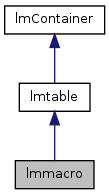[legend]
Collaboration diagram for lmmacro: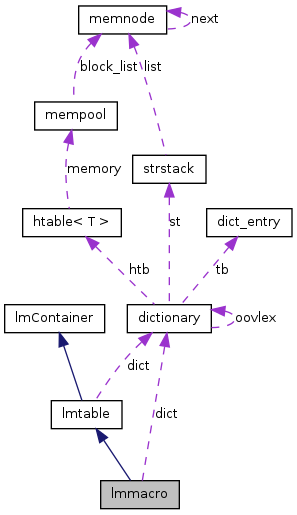[legend]

List of all members.

## Public Member Functions

lmmacro (float nlf=0.0, float dlfi=0.0)
~lmmacro ()
void load (const std::string filename, int mmap=0)
double lprob (ngram ng)
double clprob (ngram ng, double *bow=NULL, int *bol=NULL, char **maxsuffptr=NULL, unsigned int *statesize=NULL, bool *extendible=NULL)
double clprob (int *ng, int ngsize, double *bow=NULL, int *bol=NULL, char **maxsuffptr=NULL, unsigned int *statesize=NULL, bool *extendible=NULL)
const char * maxsuffptr (ngram ong, unsigned int *size=NULL)
const char * cmaxsuffptr (ngram ong, unsigned int *size=NULL)
void map (ngram *in, ngram *out)
void One2OneMapping (ngram *in, ngram *out)
void Micro2MacroMapping (ngram *in, ngram *out)
dictionarygetDict () const
int maxlevel () const
virtual void dictionary_incflag (const bool flag)
virtual bool filter (const string sfilter, lmContainer *sublmt, const string skeepunigrams)

## Detailed Description

Definition at line 40 of file lmmacro.h.

## Constructor & Destructor Documentation

 lmmacro::lmmacro ( float nlf = `0.0`, float dlfi = `0.0` )

Definition at line 53 of file lmmacro.cpp.

References getDict(), dictionary::incflag(), and NULL.

Here is the call graph for this function: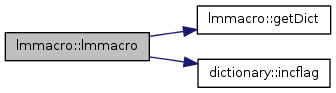lmmacro::~lmmacro ( )

Definition at line 59 of file lmmacro.cpp.

## Member Function Documentation

 double lmmacro::clprob ( int * ng, int ngsize, double * bow = `NULL`, int * bol = `NULL`, char ** maxsuffptr = `NULL`, unsigned int * statesize = `NULL`, bool * extendible = `NULL` ) ` [virtual]`

Reimplemented from lmtable.

Definition at line 324 of file lmmacro.cpp.

References clprob(), getDict(), and ngram::pushc().

Here is the call graph for this function: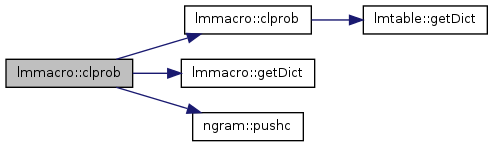double lmmacro::clprob ( ngram ng, double * bow = `NULL`, int * bol = `NULL`, char ** maxsuffptr = `NULL`, unsigned int * statesize = `NULL`, bool * extendible = `NULL` ) ` [virtual]`

Reimplemented from lmtable.

Definition at line 331 of file lmmacro.cpp.

References lmtable::getDict(), and VERBOSE.

Referenced by clprob().

Here is the call graph for this function: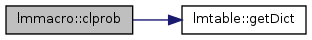Here is the caller graph for this function: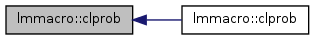const char * lmmacro::cmaxsuffptr ( ngram ong, unsigned int * size = `NULL` ) ` [virtual]`

Reimplemented from lmtable.

Definition at line 536 of file lmmacro.cpp.

References ngram::dict, lmtable::getDict(), map(), ngram::trans(), and VERBOSE.

Here is the call graph for this function: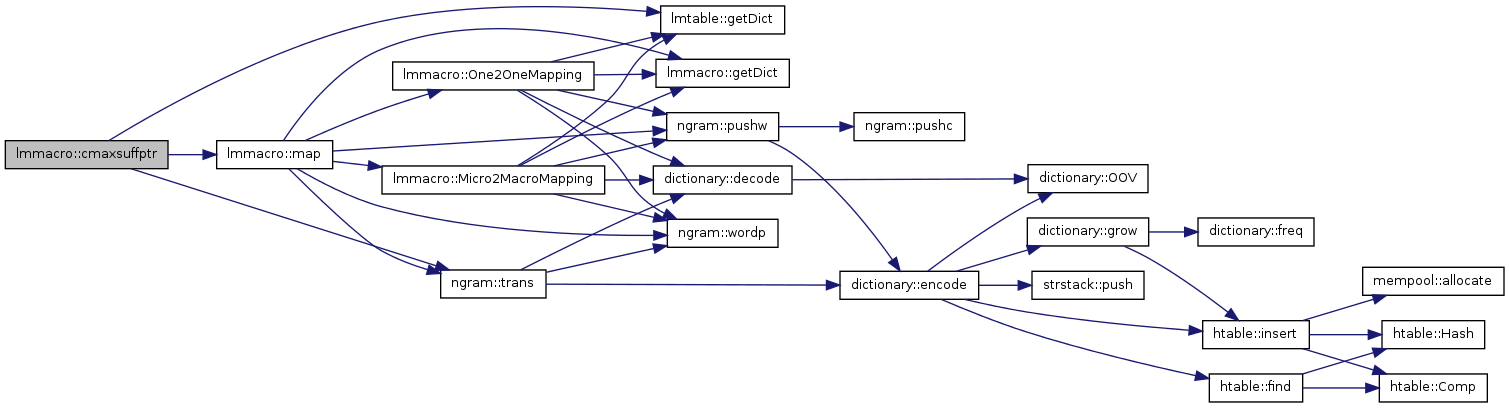virtual void lmmacro::dictionary_incflag ( const bool flag ) ` [inline, virtual]`

Reimplemented from lmtable.

Definition at line 101 of file lmmacro.h.

References dictionary::incflag().

Here is the call graph for this function: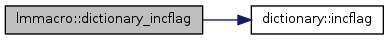virtual bool lmmacro::filter ( const string sfilter, lmContainer * sublmt, const string skeepunigrams ) ` [inline, virtual]`

Definition at line 105 of file lmmacro.h.

References UNUSED.

 dictionary* lmmacro::getDict ( ) const` [inline, virtual]`

Reimplemented from lmtable.

Definition at line 94 of file lmmacro.h.

Referenced by clprob(), lmmacro(), load(), map(), Micro2MacroMapping(), and One2OneMapping().

Here is the caller graph for this function: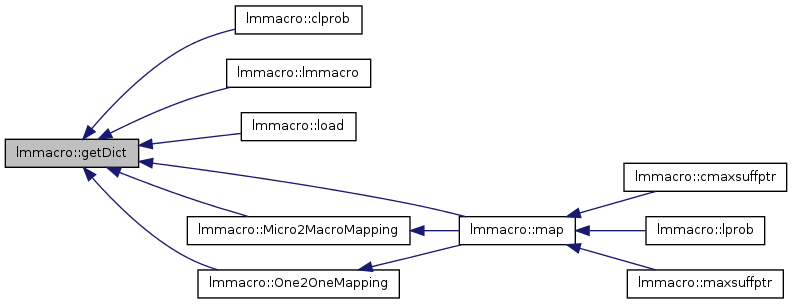void lmmacro::load ( const std::string filename, int mmap = `0` ) ` [virtual]`

Reimplemented from lmtable.

Definition at line 65 of file lmmacro.cpp.

Here is the call graph for this function: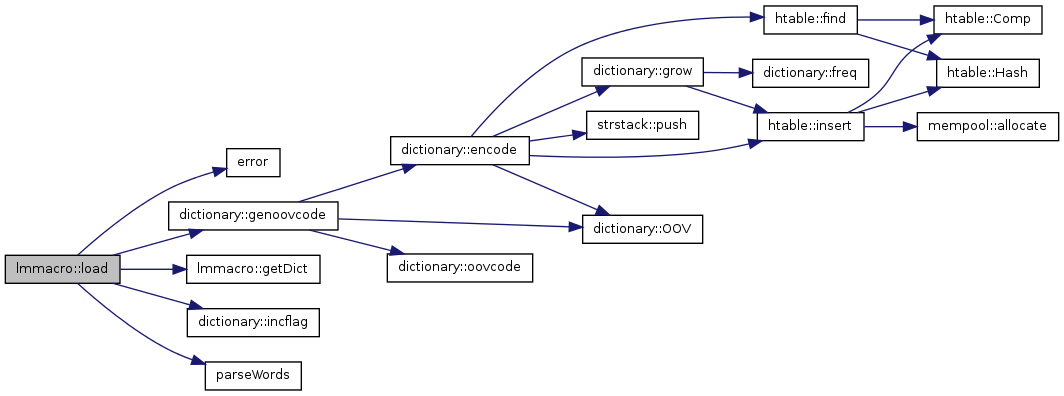double lmmacro::lprob ( ngram ng )

Definition at line 302 of file lmmacro.cpp.

References ngram::dict, lmtable::getDict(), map(), lmtable::prob(), ngram::trans(), and VERBOSE.

Here is the call graph for this function: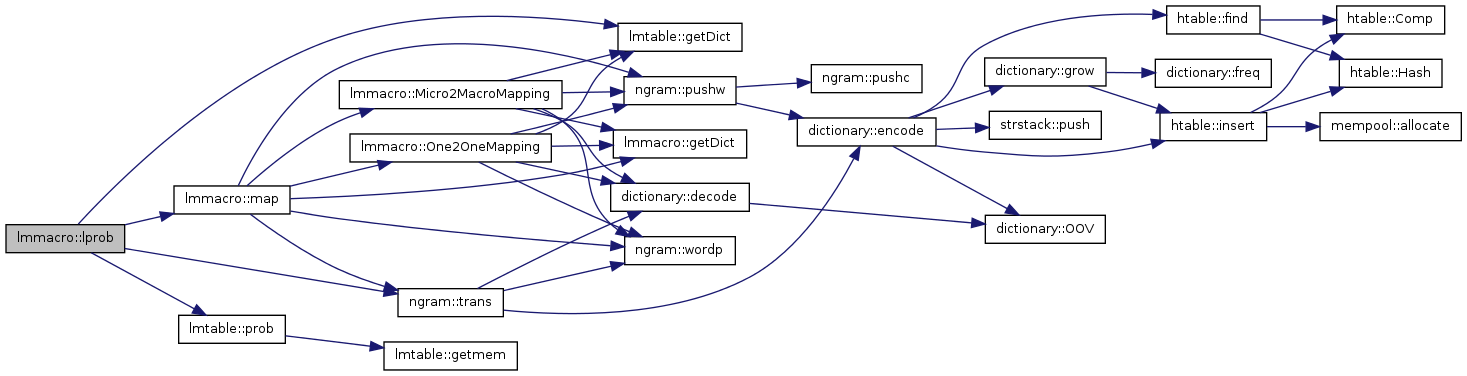void lmmacro::map ( ngram * in, ngram * out )

We can be here in 2 cases:

a. effectively when the token is malformed, that is the selected field does not exist

b. in case of verbatim translation, that is the source word is not known to the phrase table and moses transfers it as it is to the target side: in this case, no assumption can be made on its format, which means that the selected field can not exist

The possibility of case (b) makes incorrect the error exit from the code at this point: correct, on the contrary, push the _unk_ string

Definition at line 561 of file lmmacro.cpp.

Referenced by cmaxsuffptr(), lprob(), and maxsuffptr().

Here is the call graph for this function: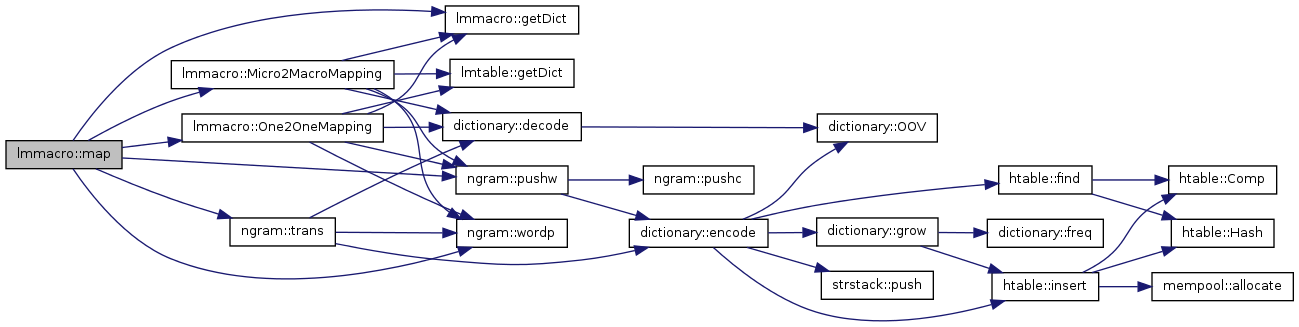Here is the caller graph for this function: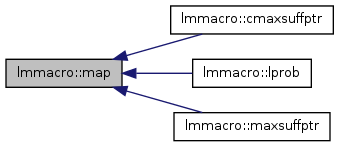int lmmacro::maxlevel ( ) const` [inline, virtual]`

Reimplemented from lmtable.

Definition at line 97 of file lmmacro.h.

 const char * lmmacro::maxsuffptr ( ngram ong, unsigned int * size = `NULL` ) ` [virtual]`

Reimplemented from lmtable.

Definition at line 521 of file lmmacro.cpp.

References ngram::dict, lmtable::getDict(), map(), ngram::trans(), and VERBOSE.

Here is the call graph for this function: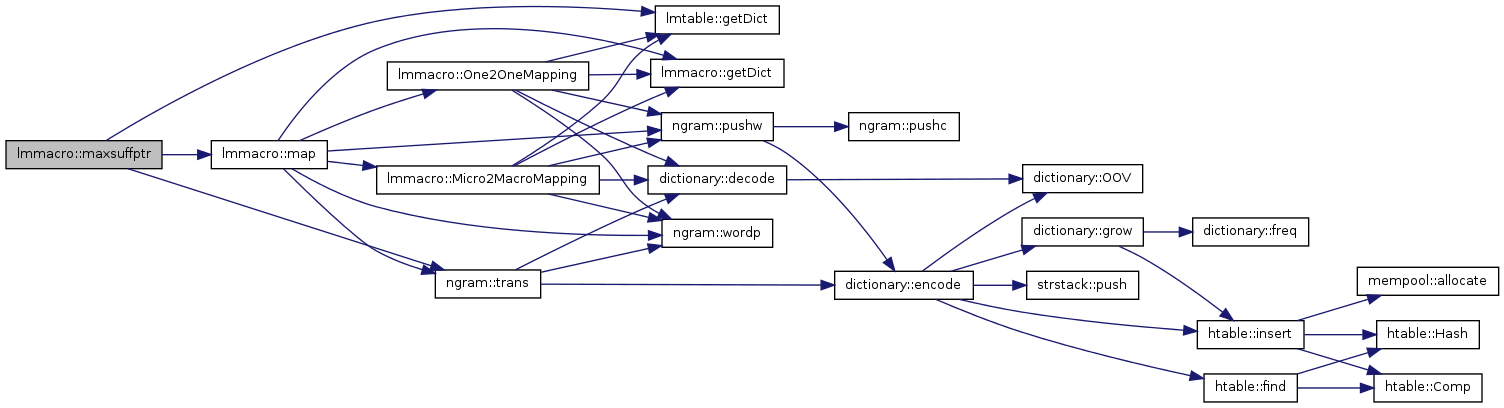void lmmacro::Micro2MacroMapping ( ngram * in, ngram * out )

Definition at line 706 of file lmmacro.cpp.

Referenced by map().

Here is the call graph for this function: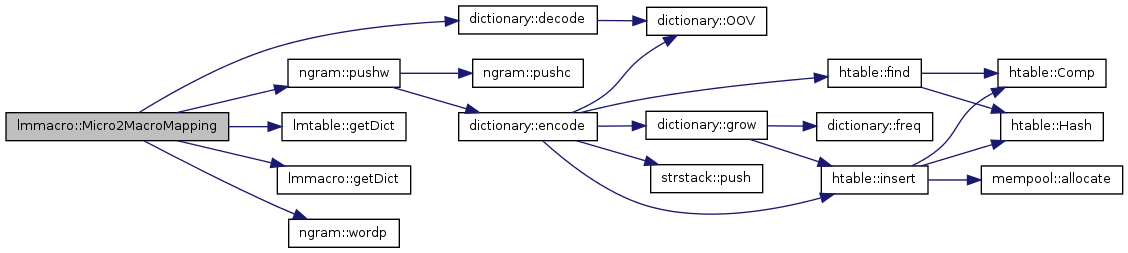Here is the caller graph for this function: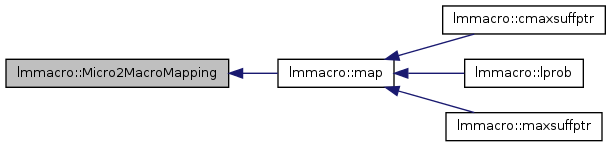void lmmacro::One2OneMapping ( ngram * in, ngram * out )

Definition at line 689 of file lmmacro.cpp.

Referenced by map().

Here is the call graph for this function: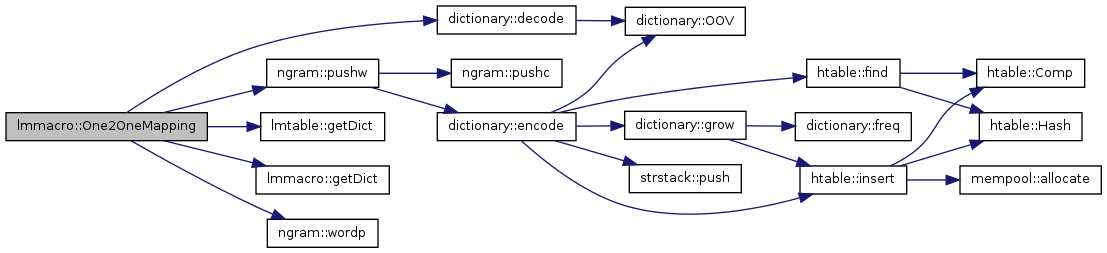Here is the caller graph for this function: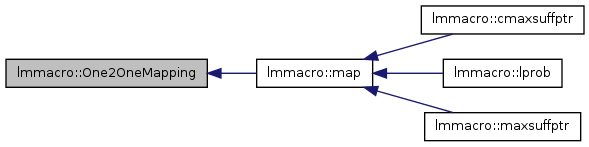The documentation for this class was generated from the following files:
• /disk4/html/www/moses/doxygen/irstlm/trunk/src/lmmacro.h
• /disk4/html/www/moses/doxygen/irstlm/trunk/src/lmmacro.cpp

Generated on Thu Jul 6 00:31:49 2017 for Moses by1.5.9